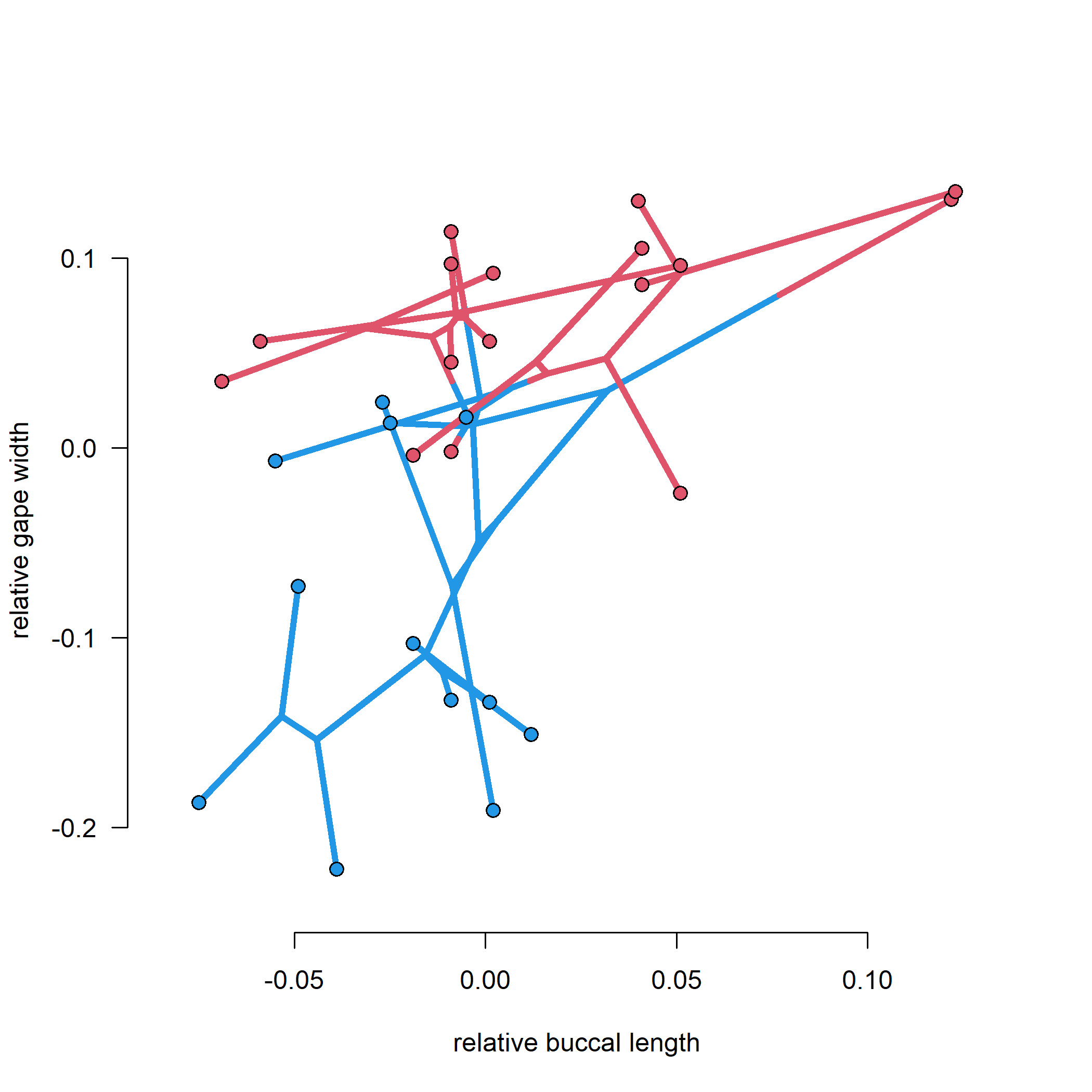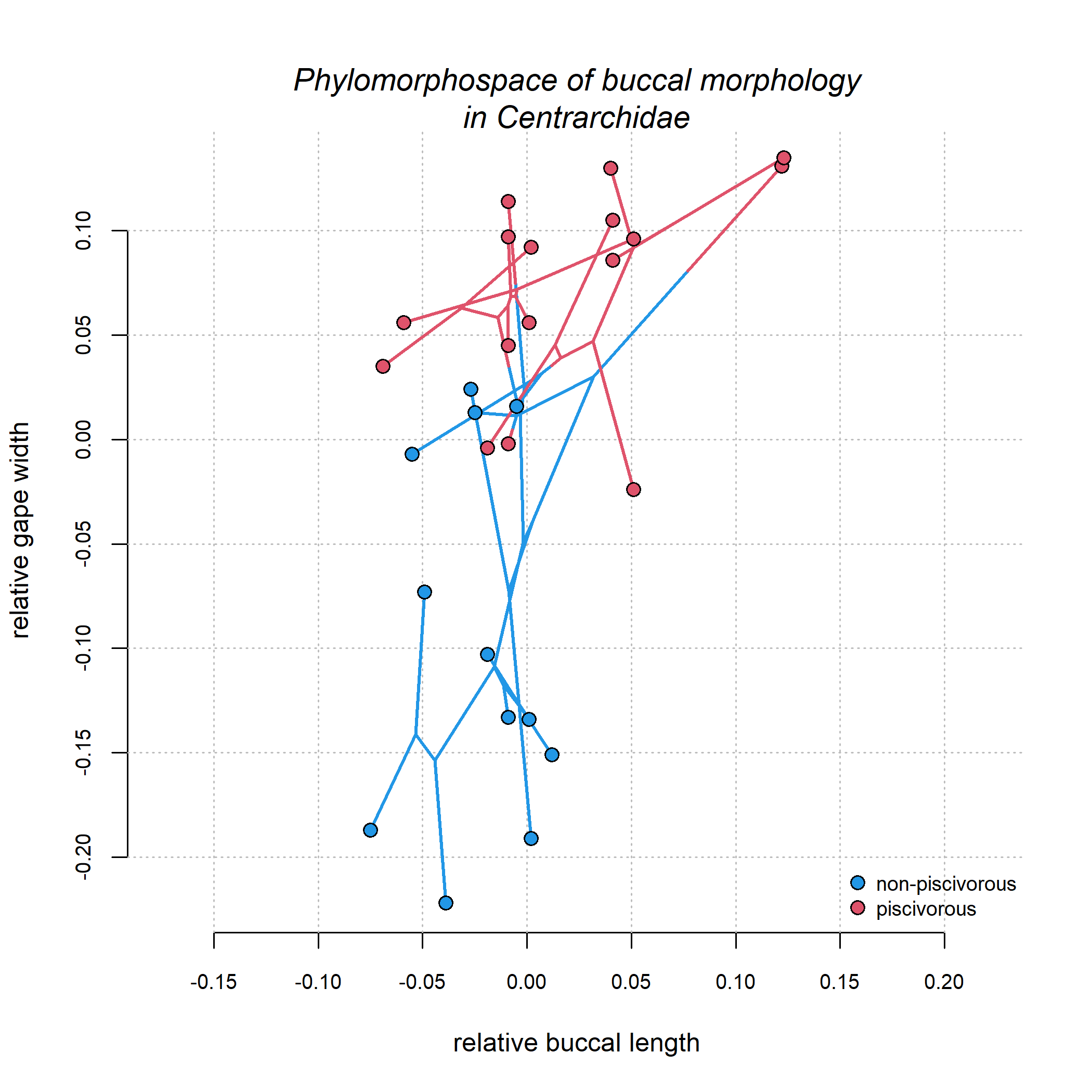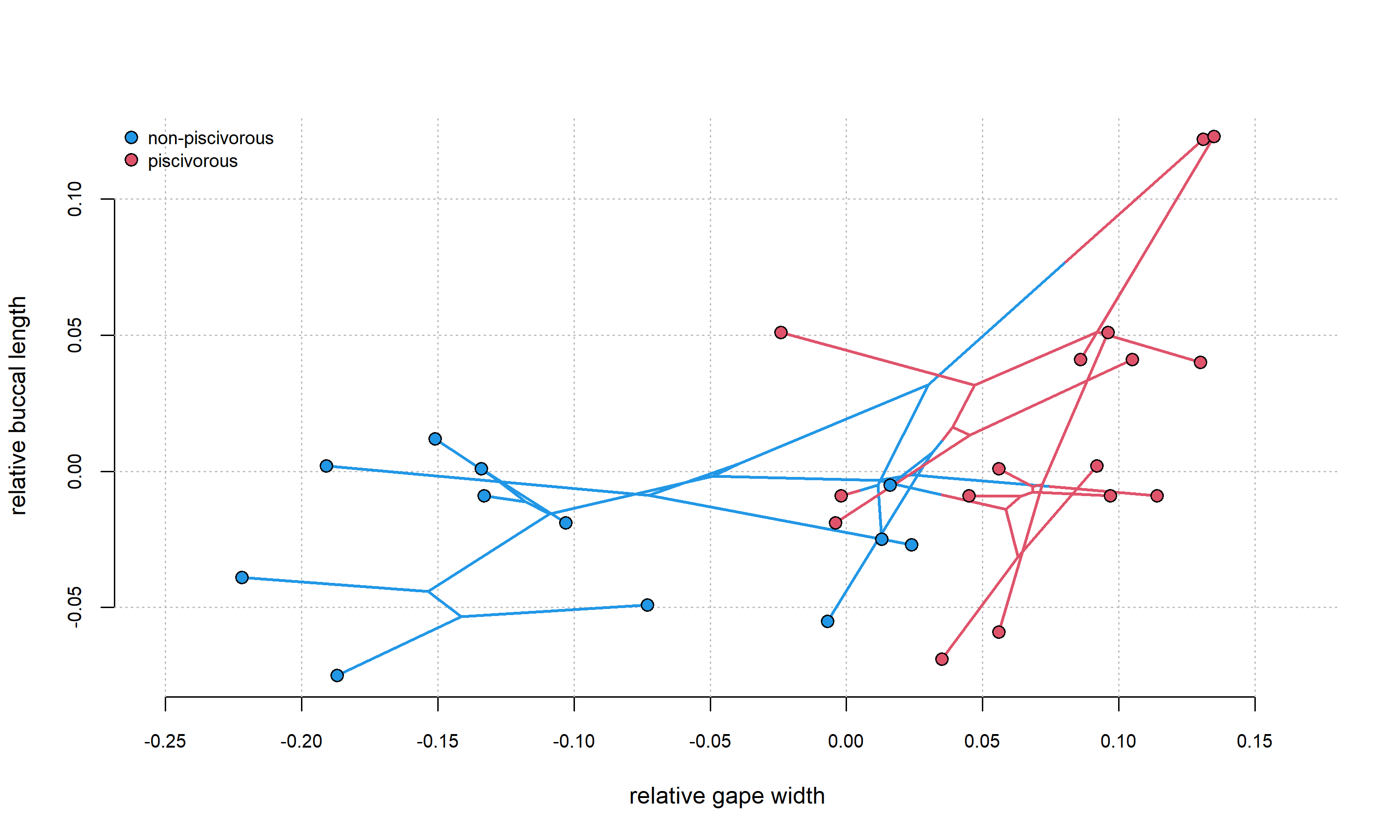## Friday, October 28, 2022

### Phylomorphospace projection with a fixed aspect ratio

Normally, the argument `asp` can be used to set the aspect ratio (basically, the relationship between real width and length in our plotting device and the units of the x and y variables that are being plotted.

Unfortunately, `phylomorphospace` does not allow the user to supply an argument for `asp`, nor can `asp` be set using `graphics::par`.

Fortunately, an easy work-around exists and that involves first opening a plotting device and setting `asp` to the desired value (the most common non-default value of `asp` desired by users is `asp = 1`), and then using the `phylomorphospace` argument `add=TRUE` to add our tree projection to the open device.

First, we can see the default plot and how that looks. For this example, I'll use a tree & dataset that comes from a study by Dave Collar & I (Revell & Collar 2009).

``````data(sunfish.tree)
data(sunfish.data)
cols<-setNames(palette()[c(4,2)],
levels(sunfish.data\$feeding.mode))
phylomorphospace(sunfish.tree,sunfish.data[,3:2],
bty="n",colors=cols,lwd=4,
ftype="off",node.by.map=TRUE,
node.size=c(0,1.2),
xlab="relative buccal length",
ylab="relative gape width",las=1)
``````Next, let's redo this but setting `asp=1`. To do that, I'll first open an empty plot with `asp=1`, then I'll add my phylomorphospace projection to that device.

While I'm at it I'll also show how to add a background grid to the plot.

``````plot(NA,xlim=range(sunfish.data[,3]),
ylim=range(sunfish.data[,2]),asp=1,
axes=FALSE,xlab="relative buccal length",
ylab="relative gape width")
axis(1,at=round(seq(-0.15,0.2,by=0.05),2),
cex.axis=0.8)
axis(2,at=seq(-0.2,0.1,by=0.05),cex.axis=0.8)
abline(v=seq(-0.25,0.25,by=0.05),col="grey",
lty="dotted")
abline(h=seq(-0.25,0.25,by=0.05),col="grey",
lty="dotted")
phylomorphospace(sunfish.tree,
sunfish.data[,3:2],colors=cols,lwd=2,
ftype="off",node.by.map=TRUE,
legend("bottomright",c("non-piscivorous","piscivorous"),
pch=21,pt.bg=cols,bty="n",pt.cex=1.2,cex=0.8)
title(main="Phylomorphospace of buccal morphology\nin Centrarchidae",
font.main=3,line=0)
``````The grid helps us see that we succeeded in setting `asp=1` since our grid lines (which are spaced evenly) form perfect squares in our plot.

As an addendum, it is not necessary to turn of axes (`axes=FALSE`) and then add the x and y axes separately using `axis` unless you want to specify the locations of the tick-marks, as I decided to do here.

That's OK, though.

Lastly, since our data (when graphed this way) are taller than wide, let's flip the x and y axes, widen our plotting device, but still maintain `asp=1`.

``````plot(NA,xlim=range(sunfish.data[,2]),
ylim=range(sunfish.data[,3]),asp=1,
axes=FALSE,xlab="relative gape width",
ylab="relative buccal length")
axis(1,at=round(seq(-0.25,0.15,by=0.05),2),
cex.axis=0.8)
axis(2,at=seq(-0.05,0.1,by=0.05),cex.axis=0.8)
abline(v=seq(-0.25,0.25,by=0.05),col="grey",
lty="dotted")
abline(h=seq(-0.25,0.25,by=0.05),col="grey",
lty="dotted")
phylomorphospace(sunfish.tree,
sunfish.data[,2:3],colors=cols,lwd=2,
ftype="off",node.by.map=TRUE,